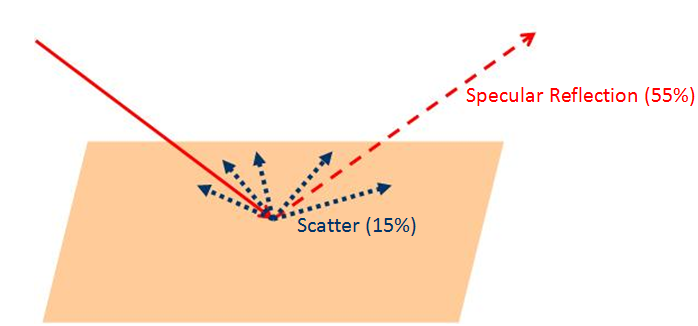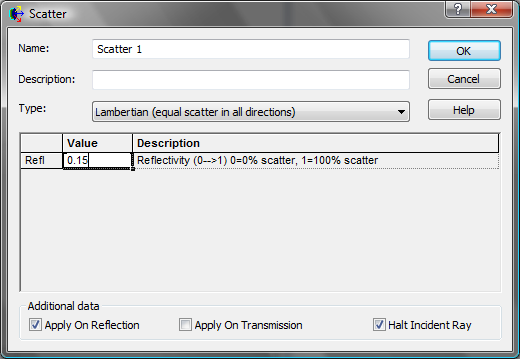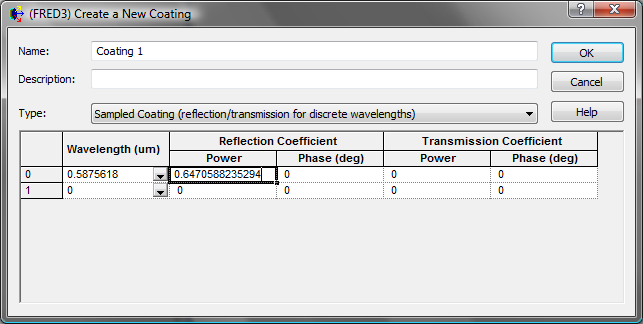# Combining Scatter with Coatings

During a raytracing process, rays encounter surfaces that might have a combination of reflection, transmission, absorption and scatter properties that split the rays into various components.

FRED allocates flux values to each ray by looking at these surface properties in a specific order. The user needs to understand this order to be certain that the actual flux values created during the raytrace are as intended. This is demonstrated by considering a surface that has Lambertian scatter properties along with a user-defined specular reflection coefficient.

Depending on the assigned models, a ray incident on a surface could be split into specular reflected rays, specular transmitted rays, scattered reflected rays, scattered transmitted rays and absorbed rays. During this process, conservation of energy dictates that the sum of these transmitted, reflected and absorbed components is equal to the incident power flux.

Ftrans_spec + Ftrans_scat + Frefl_spec + Frefl_scat + Fabs = Finc

FRED assigns the flux for each component of the above equation in a specific sequence.  When a user wishes to define surfaces that have both scatter properties and reflection / transmission coatings assigned, knowing this order is critical to correctly defining the power coefficients for the individual components.

The order that FRED follows during this power allocation is as follows:

1.      FRED determines the flux in the reflected scattered rays:

Frefl_scat = TISrefl * Finc                                        (a)

2.      FRED determines the flux in the transmitted scattered rays:

Ftrans_scat = TIStrans * Finc                                               (b)

3.      FRED determines the flux in the reflected specular rays:

Frefl = (1 – TISrefl – TIStrans) * R * Finc                     (c)

4.      FRED determines the flux in the transmitted specular rays:

Ftrans = (1 – TISrefl – TIStrans) * T * Finc                   (d)

5.      FRED attributes any remaining flux to be “absorbed”:

Fabs = Finc – TISrefl – TIStrans – Frefl - Ftrans           (e)

Note: TISrefl and TIStrans are the Total Integrated Scatter values of the reflected and transmitted scattering respectively.

Example

The significance of this sequence is shown in a simple example where the aim is to define a surface that has the following properties:

• a simple scatter model that defines 15% reflectance in a Lambertian profile
• a reflection coating that defines 55% specular reflection.
• the remaining 30% power is absorbed.Assigning a new Lambertian scatter model and defining the TIS is straightforward. In this case the Refl coefficient is set to be 0.15 I.e. 15% of incident power. This is the TISrefl component discussed above.Defining the reflected flux takes a little more care. Since the aim is to define a surface that reflects 55% of the incident power in the specular direction it might be assumed that setting the reflection coefficient ( R) of the coating to be 0.55 would be sufficient. However this is not correct and will actually define a model that has a specular reflection value 46.75%.

This is because FRED has already allocated 15% of the incident flux to the scatter model. The reflection coefficient of 0.55 is actually applied to the remaining power after the scattering is taken into account.

55% * (1 – 0.15) = 46.75%

To obtain a reflected scatter flux of 55% * Finc the reflection coefficient (R) of the coating is determined by equation (c) above and in this case needs to be:

Frefl = (1 – TISrefl) * R * Finc

0.55 = (1 – 0.15) * R

Thus R needs to be 0.6470588235294.